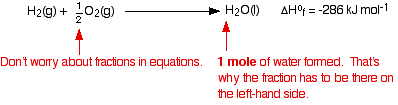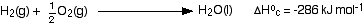# Assorted Definitions

$$\newcommand{\vecs}{\overset { \rightharpoonup} {\mathbf{#1}} }$$ $$\newcommand{\vecd}{\overset{-\!-\!\rightharpoonup}{\vphantom{a}\smash {#1}}}$$$$\newcommand{\id}{\mathrm{id}}$$ $$\newcommand{\Span}{\mathrm{span}}$$ $$\newcommand{\kernel}{\mathrm{null}\,}$$ $$\newcommand{\range}{\mathrm{range}\,}$$ $$\newcommand{\RealPart}{\mathrm{Re}}$$ $$\newcommand{\ImaginaryPart}{\mathrm{Im}}$$ $$\newcommand{\Argument}{\mathrm{Arg}}$$ $$\newcommand{\norm}{\| #1 \|}$$ $$\newcommand{\inner}{\langle #1, #2 \rangle}$$ $$\newcommand{\Span}{\mathrm{span}}$$ $$\newcommand{\id}{\mathrm{id}}$$ $$\newcommand{\Span}{\mathrm{span}}$$ $$\newcommand{\kernel}{\mathrm{null}\,}$$ $$\newcommand{\range}{\mathrm{range}\,}$$ $$\newcommand{\RealPart}{\mathrm{Re}}$$ $$\newcommand{\ImaginaryPart}{\mathrm{Im}}$$ $$\newcommand{\Argument}{\mathrm{Arg}}$$ $$\newcommand{\norm}{\| #1 \|}$$ $$\newcommand{\inner}{\langle #1, #2 \rangle}$$ $$\newcommand{\Span}{\mathrm{span}}$$$$\newcommand{\AA}{\unicode[.8,0]{x212B}}$$

This page explains what an enthalpy change is, and then gives a definition and brief comment for three of the various kinds of enthalpy change that you will come across.

## Enthalpy changes

Enthalpy change is the name given to the amount of heat evolved or absorbed in a reaction carried out at constant pressure. It is given the symbol ΔH, read as "delta H".

## Standard enthalpy changes

Standard enthalpy changes refer to reactions done under standard conditions, and with everything present in their standard states. Standard states are sometimes referred to as "reference states".

### Standard conditions

Standard conditions are:

• 298 K (25°C)
• a pressure of 1 bar (100 kPa).
• where solutions are involved, a concentration of 1 mol dm-3

### Standard states

For a standard enthalpy change everything has to be present in its standard state. That is the physical and chemical state that you would expect to find it in under standard conditions.

That means that the standard state for water, for example, is liquid water, H2O(l) - not steam or water vapour or ice.

Oxygen's standard state is the gas, O2(g) - not liquid oxygen or oxygen atoms.

For elements which have allotropes (two different forms of the element in the same physical state), the standard state is the most energetically stable of the allotropes.

For example, carbon exists in the solid state as both diamond and graphite. Graphite is energetically slightly more stable than diamond, and so graphite is taken as the standard state of carbon.

Similarly, under standard conditions, oxygen can exist as O2 (simply called oxygen) or as O3 (called ozone - but it is just an allotrope of oxygen). The O2 form is far more energetically stable than O3, so the standard state for oxygen is the common O2(g).

## The symbol for standard enthalpy changes

The symbol for a standard enthalpy change is ΔH°, read as "delta H standard" or, perhaps more commonly, as "delta H nought".

## Standard enthalpy change of reaction, ΔH°r

Remember that an enthalpy change is the heat evolved or absorbed when a reaction takes place at constant pressure. The standard enthalpy change of a reaction is the enthalpy change which occurs when equation quantities of materials react under standard conditions, and with everything in its standard state.

That needs exploring a bit. Here is a simple reaction between hydrogen and oxygen to make water:• First, notice that the symbol for a standard enthalpy change of reaction is ΔH°r. For enthalpy changes of reaction, the "r" (for reaction) is often missed off - it is just assumed.
• The "kJ mol-1" (kilojoules per mole) doesn't refer to any particular substance in the equation. Instead it refers to the quantities of all the substances given in the equation. In this case, 572 kJ of heat is evolved when 2 moles of hydrogen gas react with 1 mole of oxygen gas to form 2 moles of liquid water.
• Notice that everything is in its standard state. In particular, the water has to be formed as a liquid.
• And there is a hidden problem! The figure quoted is for the reaction under standard conditions, but hydrogen and oxygen don't react under standard conditions.

Whenever a standard enthalpy change is quoted, standard conditions are assumed. If the reaction has to be done under different conditions, a different enthalpy change would be recorded. That has to be calculated back to what it would be under standard conditions. Fortunately, you don't have to know how to do that at this level.

## Standard enthalpy change of formation, ΔH°f

The standard enthalpy change of formation of a compound is the enthalpy change which occurs when one mole of the compound is formed from its elements under standard conditions, and with everything in its standard state.

The equation showing the standard enthalpy change of formation for water is:When you are writing one of these equations for enthalpy change of formation, you must end up with 1 mole of the compound. If that needs you to write fractions on the left-hand side of the equation, that is OK. (In fact, it is not just OK, it is essential, because otherwise you will end up with more than 1 mole of compound, or else the equation won't balance!)

The equation shows that 286 kJ of heat energy is given out when 1 mole of liquid water is formed from its elements under standard conditions.

Standard enthalpy changes of formation can be written for any compound, even if you can't make it directly from the elements. For example, the standard enthalpy change of formation for liquid benzene is +49 kJ mol-1. The equation is:If carbon won't react with hydrogen to make benzene, what is the point of this, and how does anybody know what the enthalpy change is?

What the figure of +49 shows is the relative positions of benzene and its elements on an energy diagram:How do we know this if the reaction doesn't happen? It is actually very simple to calculate it from other values which we can measure - for example, from enthalpy changes of combustion (coming up next). We will come back to this again when we look at calculations on another page.

Knowing the enthalpy changes of formation of compounds enables you to calculate the enthalpy changes in a whole host of reactions and, again, we will explore that in a bit more detail on another page.

And one final comment about enthalpy changes of formation:

The standard enthalpy change of formation of an element in its standard state is zero. That's an important fact. The reason is obvious . . .

For example, if you "make" one mole of hydrogen gas starting from one mole of hydrogen gas you aren't changing it in any way, so you wouldn't expect any enthalpy change. That is equally true of any other element. The enthalpy change of formation of any element has to be zero because of the way enthalpy change of formation is defined.

## Standard enthalpy change of combustion, ΔH°c

The standard enthalpy change of combustion of a compound is the enthalpy change which occurs when one mole of the compound is burned completely in oxygen under standard conditions, and with everything in its standard state.

The enthalpy change of combustion will always have a negative value, of course, because burning always releases heat. Two examples:Notice:

• Enthalpy of combustion equations will often contain fractions, because you must start with only 1 mole of whatever you are burning.
• If you are talking about standard enthalpy changes of combustion, everything must be in its standard state. One important result of this is that any water you write amongst the products must be there as liquid water.

Similarly, if you are burning something like ethanol, which is a liquid under standard conditions, you must show it as a liquid in any equation you use.

• Notice also that the equation and amount of heat evolved in the hydrogen case is exactly the same as you have already come across further up the page. At that time, it was illustrating the enthalpy of formation of water. That can happen in some simple cases. Talking about the enthalpy change of formation of water is exactly the same as talking about the enthalpy change of combustion of hydrogen.